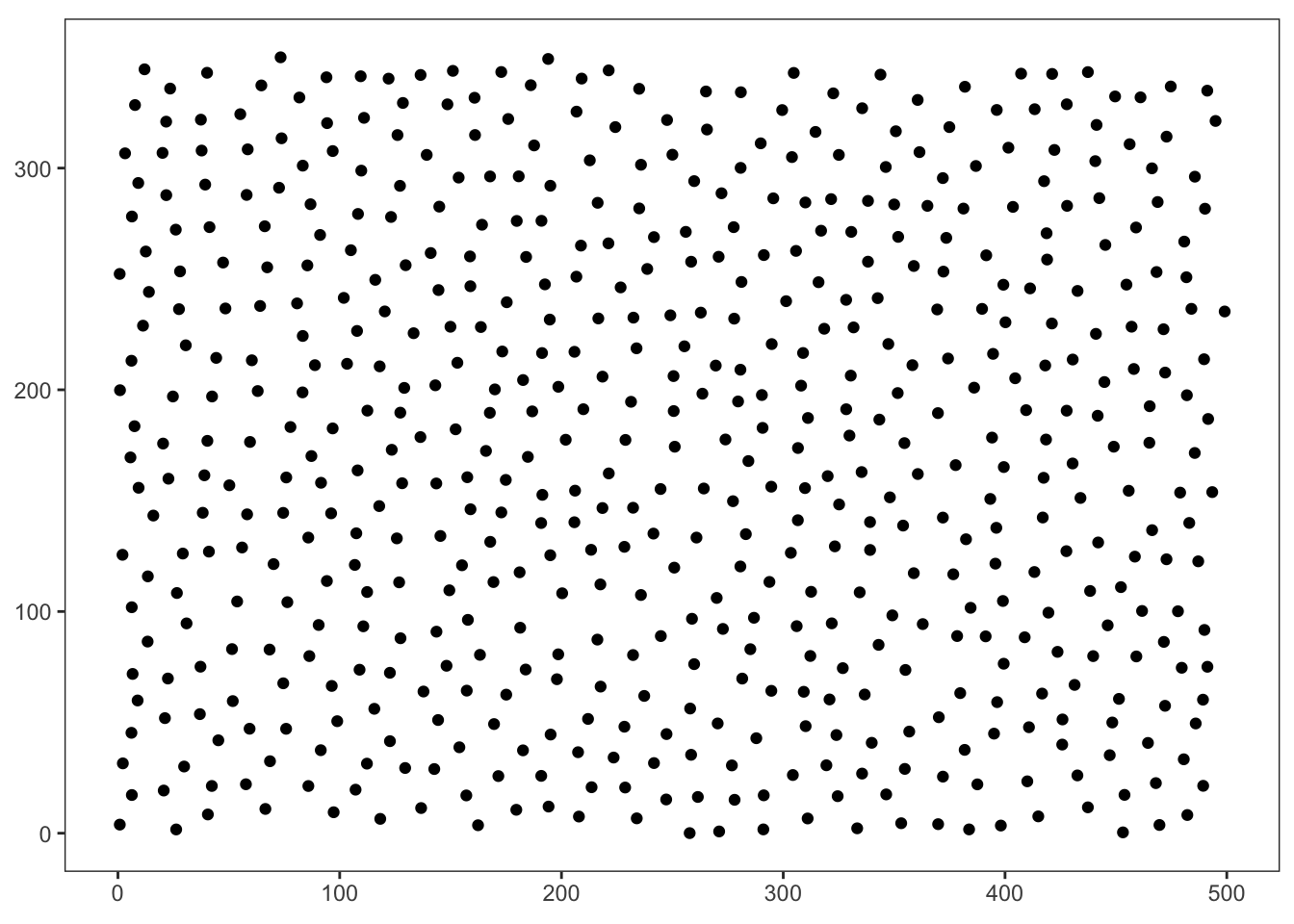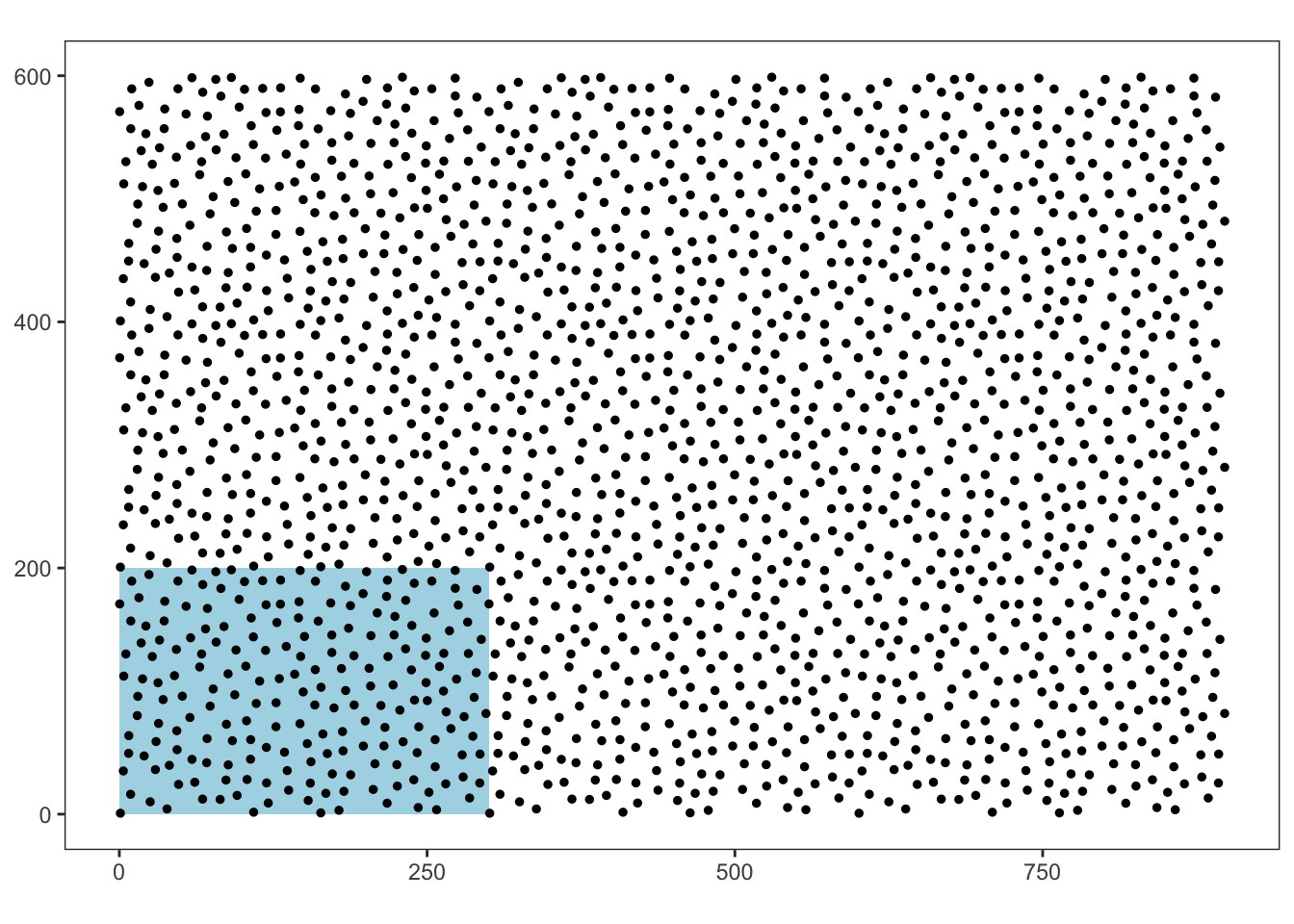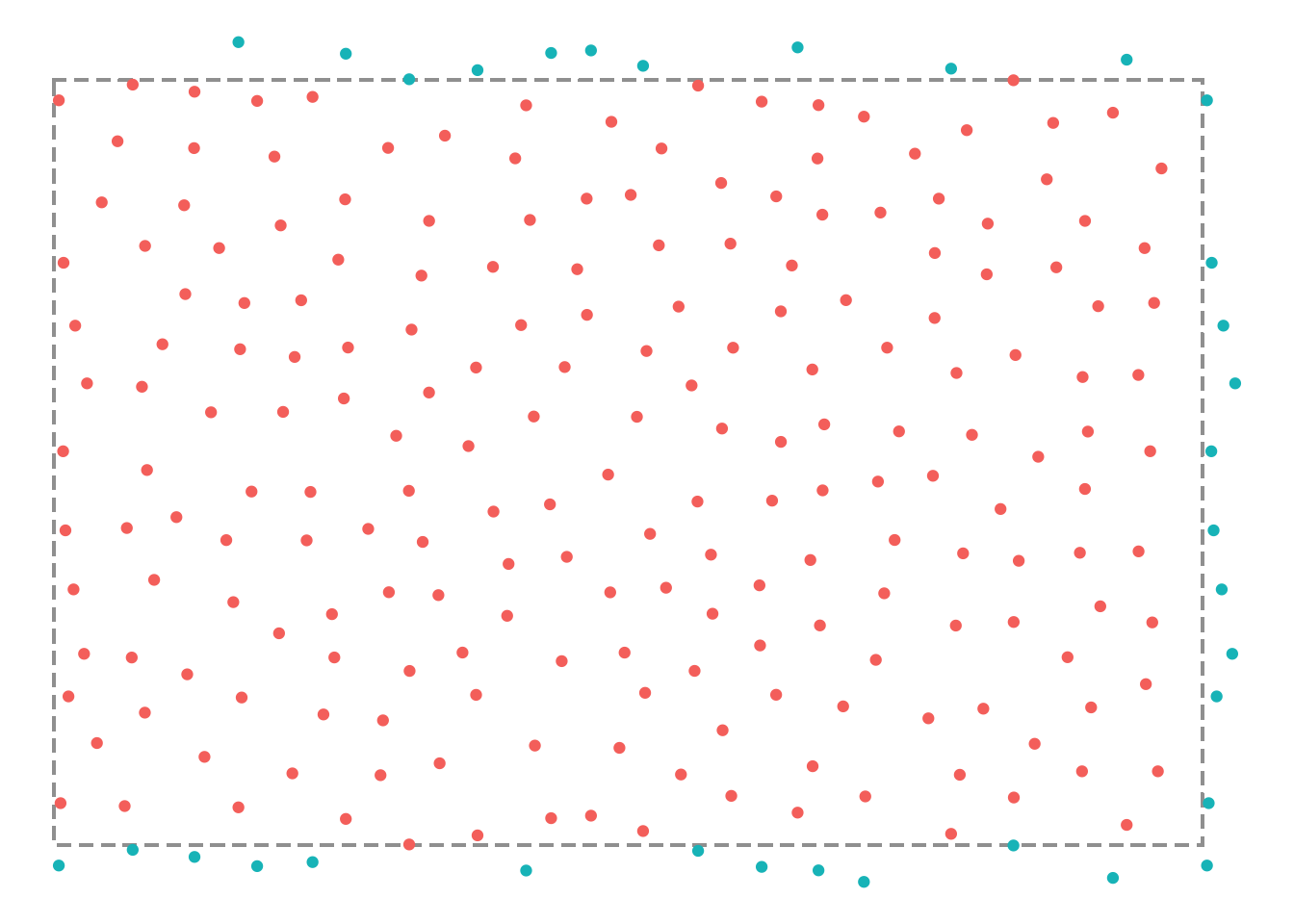# poissoned

poissoned is an Rstats implementation of the poisson disk sampling algorithm from Bridson’s paper - Fast Poisson Disk Sampling in Arbitrary Dimensions

Notes:

• only implemented the 2d case
• adjusted the algorithm in order to ensure repeatable/tileable samples with minimal artifacts at the joins
• similar in purpose to Will Chase’s package {poissondisc}. However, poissoned has a a completely different implementation which ends up being much faster.

## Installation

You can install from GitHub with:

# install.packages("devtools")
devtools::install_github("coolbutuseless/poissoned")

## Basic Usage

library(poissoned)

set.seed(1)
points <- poissoned::poisson_disc(ncols = 50, nrows = 35, cell_size = 10, verbose = TRUE)
poisson_disc(): 500x350, minimum distance = 14.14
ggplot(points) +
geom_point(aes(x, y)) +
theme_bw() +
coord_fixed() +
theme(
panel.grid = element_blank(),
axis.title = element_blank()
)# Tileability

The points generated from poissoned::poisson_disc() are tileable in that there should be minimal artifacts at the joins.

In the graph below, the initial set of points (highlighted in blue) were manually replicated and offset eight times.# Tileability - details

Tileability is achieved by copying edge points to outside the opposite edge - this simulates a toroidal coordinate system such that the canvas wraps around on itself.

These boundary points are retrievable by setting keep_boundary = TRUE.

In the following plot, the dashed line indicates the limits of the point set, and the blue points are transated points copied from the opposite edge.

points <- poissoned::poisson_disc(ncols = 30, nrows = 20, cell_size = 10, keep_boundary = TRUE, verbose = TRUE)
poisson_disc(): 300x200, minimum distance = 14.14
points\$boundary <- with(points, x < 0 | y < 0 | x > 300 | y > 200)

ggplot(points) +
geom_rect(xmin = 0, xmax = 300, ymin = 0, ymax = 200, fill = NA, colour = '#a0a0a0', linetype = 2) +
geom_point(aes(x, y, colour = boundary)) +
theme_void() +
coord_fixed() +
theme(legend.position = 'none')